CATEGORIES:

# Decomposition of vectors.

Theorem 1. An arbitrary vectorin the plane can be decomposed into two noncollinear vectors: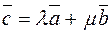.

Theorem 2. An arbitrary vectorin space can be decomposed into three noncoplanar vectors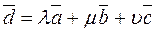.

Let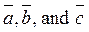be noncoplanar vectors.

The Cartesian system of coordinates. Consider the following coordinate system: take mutually perpendicular unit vectors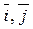and, draw coordinate axes x,y and z along them, and fix a unit on metric scale: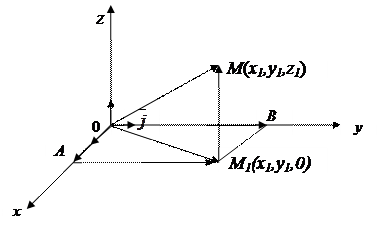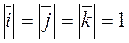,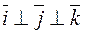.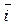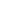Definition. The triple of vectors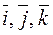is called right if, looking from the endpoint of the last vector, we see that the shorter rotation from the first vector to the second is anticlockwise.

From the triangle ОММ1, we obtain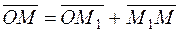,

Since the vector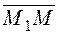is collinear to the unit vector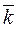, it follows that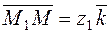.

From the triangle ОАМ1, we obtain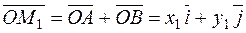,

because, by analogy, the vectorsand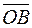are collinear to the unit vectors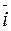and. Substituting the vector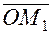thus obtained, we see that. (2)

Thus, the radius vector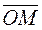is represented as the sum of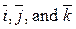and multiplied by the corresponding coordinates of the point М.

Consider the vectors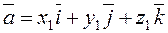andand their sum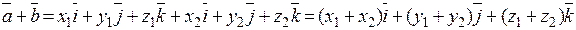.

Let us multiply the vector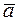by a number l: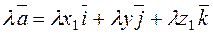.

When a vector is multiplied by a number l, each coordinate of this vector is multiplied by this number.

Example. Find the vector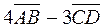if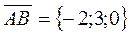;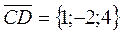.

Let us find the required vector in vector notation: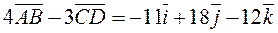.

To find the same vector in vector notation, we multiply the first vector by 4 and the second by –3 and sum their coordinates: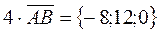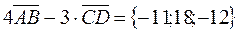.

Given two points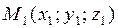and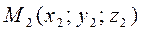in space, find the vector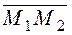.

Thus, we have found the required vector in the coordinate notation: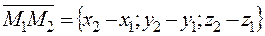. (2¢¢)

To find the coordinates of a vector, we must subtract the coordinates of its tail from the coordinates of the head.

For example, let us find vectors with given coordinates of heads and tails:

М1(7;4;–3); М2(1;–2;–2);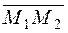={–6; –6; 1};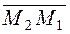={6; 6; –1}.

Find the length of a vector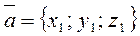: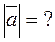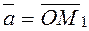.

From the right triangle ОМ1М2 , we find the hypotenuse,z

where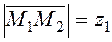.

M1

From the other right triangle ОАМ2 , 0 z1 y

we find the hypotenuse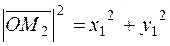.

Substituting it into А x1

the first hypotenuse, we obtain x y1 M2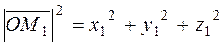.

Thus, the length of a vector is defined by the formula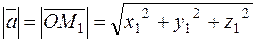. (3)

Date: 2015-01-02; view: 996

 <== previous page | next page ==> Linear Operations on Vectors | The direction of a vector. Let us find the angle between two vectors and .
doclecture.net - lectures - 2014-2021 year. Copyright infringement or personal data (0.005 sec.)